## Memory Usage

Each mode uses a different amount of memory for storing traffic data. This appendix is intended to give the user an approximation of how long a Phoenix MDK Field Unit may collect data before retrieval must be performed. Note that this is only a guide – it is recommended to retrieve data as often as is practical from the units, and deleting retrieved files from the memory (unless Erase First File When No Mem is enabled). Different configurations may store different amounts of data. For example, the amount of memory to store a single 2 axle vehicle in Raw (Per-Vehicle) Mode is 7 bytes if you are using axle sensors or presence sensors (loops). A 5 axle vehicle in the same mode will take 13 bytes if you are using axle sensors, but still 7 bytes if you are using presence (loop) sensors (the individual axle lengths are not stored since loops cannot see the individual axles).

The following tables give you formulas for calculating how much memory any particular storage mode might take. Simply find the table that most closely matches your application, and follow the steps described.

Appendix B.1 Raw (Per-Vehicle) Data Collection

Raw (Per-Vehicle) Data stores each individual vehicle in memory; therefore the amount of memory used is directly dependant on how many vehicles pass the sensors. Another important factor is the number of axles per vehicle (more axles require more memory).

Generally, you can use the average of 2.75 axles per vehicle for most highways. If your site differs from this, you may with to increase or decrease the numbers given below.

To calculate how many vehicles you can store with the Phoenix MDK follow the below steps:

• Calculate the base average number of bytes per vehicle using the chart below:
 SENSOR CONFIGURATION NORMAL RAWDATA ENHANCED RAWDATA RAW DATA WITH BINS ENHANCED & BINS Axle – Axle Axle – Pres – Axle Pres – Axle – Pres 8.5 12.5 13.5 17.5 Pres – Pres 7 9 12 14
• Divide by the Total memory of your counter minus 2000 (for overhead) by the base number of bytes from the chart above. The total amount of memory in your counter can be found using the Show Status option (a standard Phoenix MDK comes with 1024k, which gives about 50,000 vehicles for Normal Raw Data, Axle-Axle Sensors).Appendix B.2 Binned Data Collection

Binned Data stores data as the total number of vehicles in each bin category every record interval. The three most important factors are: Which bin categories are enabled, what the record interval lengths are, and how many lanes are enabled.

Follow the steps outlines below to calculate how long your Phoenix MDK can collect data with any given setup.

• Using the table below, calculate the base number of bytes in a single record period, for a single lane. This is done by adding up all the different modes you have enables (Axle, Speed, Length, etc.). The table gives two values, the first is the default number if you have not modified the bin table configuration, the second is formulas you can use to calculate the number if you have put in your own bin table specifications. Note that “SnMis Bins” are created if you select “View&Store” from the “SnMis Storage Mode?” question in the Configure System option.
 Axle Speed Length Gap Headway Speed x Length Speed x Axle SnMis 26 2 x Bins 32 2 x Bins 26 2 x Bins 16 2 x Bins 16 2 x Bins 416 (2xSpd Bin) x Length Bin 416 (2xSpd Bin) x Axle Bin 8
• For example, if you were collecting Axle & Speed classification, your base number of bytes would be 26+32=58 bytes.
• Take your base number of bytes and multiply it by the number of enabled lanes (including directional lanes). Thus, if you had lane 1 enabled with directional, you would have two enables lanes. This number is for the BASE NUMBER FOR ALL LANES.
• Adjust the base number for all lanes by adding 1 for each type of classification you have enabled, except Speed x Length and Speed x Axle. If you enabled Speed x Length, add in the number of Length Bins. If you enables Speed x Axle, add in the number of Axle Bins.

For example, if you enable Axle, Speed and Speed x Axle classification you would add 1+1+13+base number for all lanes = TOTAL NUMBER OF BYTES PER RCORD INTERVAL.

• The next step is to take the total number of bytes in your Phoenix MDK, subtract 2000 (for overhead), and divide it by the total number of bytes per record interval. This gives you the NUMBER OF RECORD INTERVALS THE MEMORY WILL HOLD. Total and available number of bytes (memory) in your Phoenix MDK can be determined by using the Show Status option. A standard Phoenix MDK contains 512k bytes.
• Next, multiply the total number of record intervals the memory will hold by the record interval length (in minutes). This gives you the total length of time, in minutes, the Phoenix MDK will operate.
• You are basically done. You can calculate number of hours by dividing the time in minutes by 60, or the number of days by dividing the time in minutes by 1440.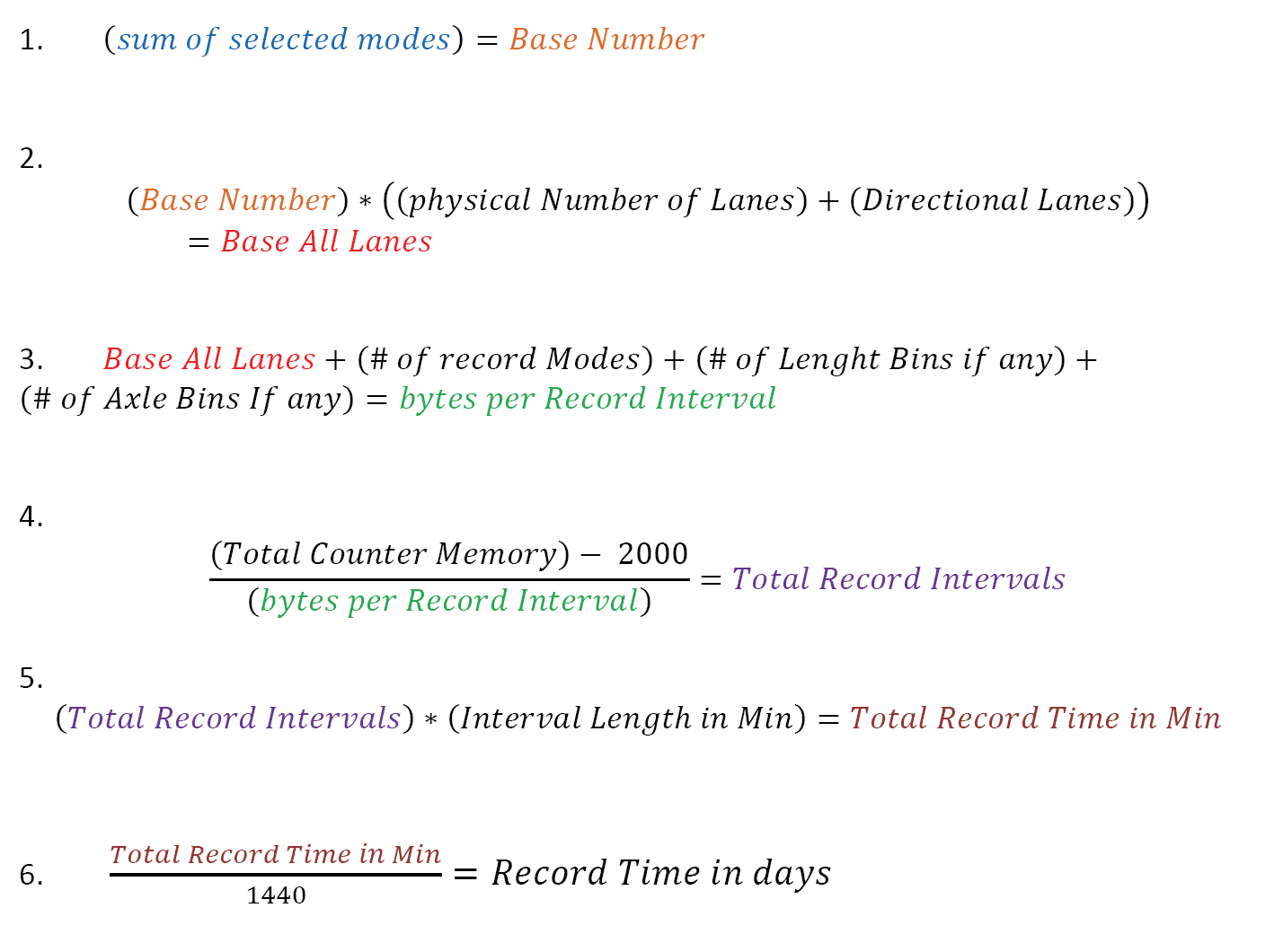Appendix B.3 Count Data Collection

Count Data stores the total number of vehicles (If using a presence sensor) or the total number of axles that crossed the sensors for a given time period. The two factors to consider are: How many lanes are enables, and what is the record interval length.

Follow the steps outlines below to calculate how long a Phoenix MDK will collect Count Data.
• Multiply the total number of enabled lanes by 2.
• Add one to the number. This is the TOTAL NUMBER OF BYTES PER RECORD INTERVAL.
• The next step is to take the total number of bytes in your Phoenix MDK, subtracting 2000 (for overhead), and divide it by the total number of bytes per record interval. This gives you the NUMBER OF RECORD INTERVALS THE MEMORY WILL HOLD. Total and available number of bytes (memory) in your Phoenix MDK can be determined by using the Show Status option. A standard Phoenix MDK contains 512k bytes.
• Next, multiply the total number of record intervals the memory will hold by the recode interval length (in minutes). This gives you the total length of time, in minutes the Phoenix MDK will operate.
• You are basically done. You can calculate number of hours by dividing the time in minutes by 60, or the number of days by dividing the time in minutes by 1440.

The above system works only if you do not have different record interval lengths during the day.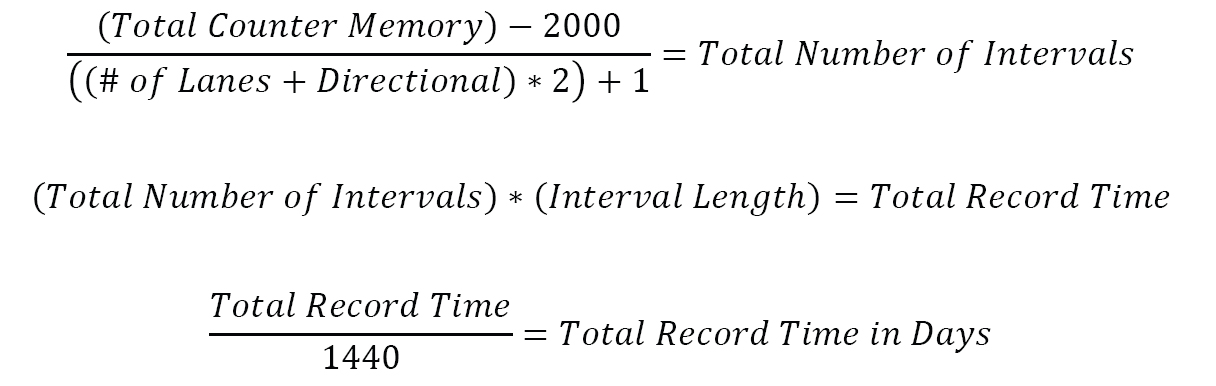Appendix B.4 Sensor Data Collection

Sensor Data storage will store in memory each individual sensor activation. Therefore, the more sensor activations you have, the quicker the memory will run out.

Follow the steps outlines below to calculate how many sensor activations can be stores in the memory of the Phoenix MDK.

• Find out the total amount of memory in the Phoenix MDK. This can be determined using the Show Status option. A standard Phoenix MDK contains 512k bytes.
• Subtract the total amount of memory 2000, for overhead.
• Divide by 8. This will be the amount of sensor activations which can be stored in memory. A standard Phoenix MDK will hold about 8300 sensor activations.### Attachments

No attachments were found.

### Article Details

Last Updated
24th of October, 2011

### Would you like to...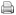Print this page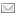Email this page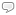Post a comment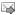Subscribe me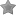Add to favorites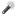Remove Highlighting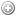Edit this ArticleQuick EditExport to PDF

### User Opinions

No users have voted.

### How would you rate this answer?

Thank you for rating this answer.